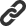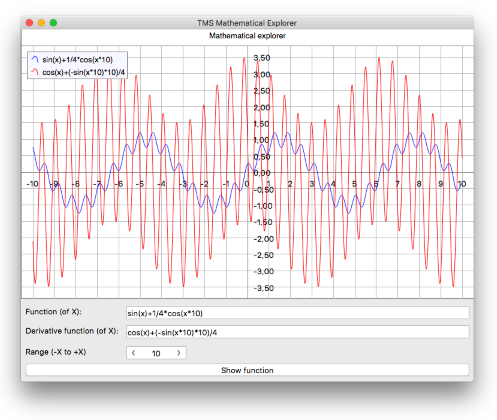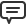# Blog

All Blog Posts  |  Next Post  |  Previous Post

#Weekend fun with Delphi, math and FNC

Bookmarks:

#### Sunday, December 11, 2016

Mathematics is fascinating, Delphi is cool, cross-platform is awesome, cross-framework is the icing on the cake. So, what's better than spending some time with all four on a weekend day?

For some time, we offer our TMS Analytics & Physics package that can do formula evaluation, complex number calculation and most interestingly also expression based derivative function calculation. Also since beginning of 2016, we offer our TMS FNC Chart, which is a chart package that offers a chart UI component that can be used in VCL applications, FMX applications and also LCL (Lazarus/FPC based) applications. This means that with the TMS FNC Chart, we can target Win32, Win64, macOS, iOS, Android, Linux, Raspbian operating systems.

So, I set out this weekend to spend some time creating a simple mathematical formula plotting application (cross platform & cross framework) that could not only display a function but also calculate its derivative function and plot it. This way, we can visually see the effect of calculating a derivative function.
The setup of this application turns out to be amazingly simple with some help of TMS FNC Chart and TMS Analytics & Physics Pack.

The core code is a model class that does chart initialization & formula calculation + derivative calculation by means of two class methods. This model can be used by the VCL, FMX or LCL application as apart from unit references, all code is framework independent. In this model, calculation is central and is nothing more than performing parsing of a mathematical formula and calculating it's derivative and Y-values for a range of X-values and display this in a chart. To do this, we initialize the TTranslator class (part of TMS Analytics) and specify the X is the main variable of the functions we can write and then we check the syntax, calculate the derivative and calculate values for both function and derivative function:
```  FTransMain := TTranslator.Create;
FTransDeriv :=  TTranslator.Create;

vName := 'x';
vValue := 1.0;

try
if FTransMain.CheckSyntax(f) then
begin
df := FTransMain.Derivative(f,'x');

if FTransDeriv.CheckSyntax(df) then
begin
for i := -xrange to +xrange do
begin
// get Y value of the function for a given X value
FTransMain.Variables['x'].Value := i;
v := FTransMain.Calculate(f);
// add the Y point to the XY chart series 1

// get Y value of the derivative function for a given X value
FTransDeriv.Variables['x'].Value := i;
v := FTransDeriv.Calculate(df);
// add the Y point to the XY chart series 2
end;
end;
end;
finally
FTransMain.Free;
FTransDeriv.Free;
end;```
This is the core code responsible for coming to a result for a function like sin(x)+1/4*cos(x*10) that you can think of as mathematical basis for modulation techniques.FMX version of the Math Explorer app running on macOS

Of course, the intention of the math exploring application is that you can enter any other mathematical function of X and not only see the derivative function but also plot it in the chart. Download & install TMS Analytics & Physics and TMS FNC Chart and then compile and run the VCL or FMX version of the math explorer application you can download with full source code.

Bruno Fierens

Bookmarks: